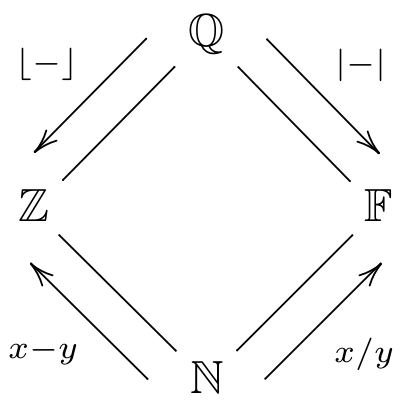# Numeric types¶

Disco has four types which represent numbers:

• Natural numbers, written N, ℕ, Nat, or Natural. These represent the counting numbers 0, 1, 2, … which can be added and multiplied.

Disco> :type 5
5 : ℕ

• Integers, written Z, ℤ, Int, or Integer, allow negative numbers such as -5. They extend the natural numbers with subtraction.

Disco> :type -5
-5 : ℤ

• Fractional numbers, written F, 𝔽, Frac, or Fractional, allow fractions like 2/3. They extend the natural numbers with division.

Disco> :type 2/3
2 / 3 : 𝔽

• Rational numbers, written Q, ℚ, or Rational, allow both negative and fractional numbers, such as -2/3.

Disco> :type -2/3
-2 / 3 : ℚ


We can arrange the four numeric types in a diamond shape, like this:Each type is a subset, or subtype, of the type or types above it. For example, the fact that $$\mathbb{N}$$ is below $$\mathbb{Z}$$ means that every natural number is also an integer.

• The values of every numeric type can be added and multiplied.
• The arrow labelled $$x-y$$ indicates that going up and to the left in the diamond (i.e. from $$\mathbb{N}$$ to Z or F to Q) corresponds to adding the ability to do subtraction. That is, values of types on the upper left of the diamond ($$\mathbb{Z}$$ and $$\mathbb{Q}$$) can also be subtracted.
• Going up and to the right corresponds to adding the ability to do division; that is, values of the types on the upper right of the diamond ($$\mathbb{F}$$ and $$\mathbb{Q}$$) can also be divided.
• To move down and to the right (i.e. from $$\mathbb{Z}$$ to $$\mathbb{N}$$, or from $$\mathbb{Q}$$ to $$\mathbb{F}$$), you can use absolute value.
• To move down and to the left (i.e. from $$\mathbb{F}$$ to $$\mathbb{N}$$, or from $$\mathbb{Q}$$ to $$\mathbb{Z}$$), you can take the floor or ceiling.